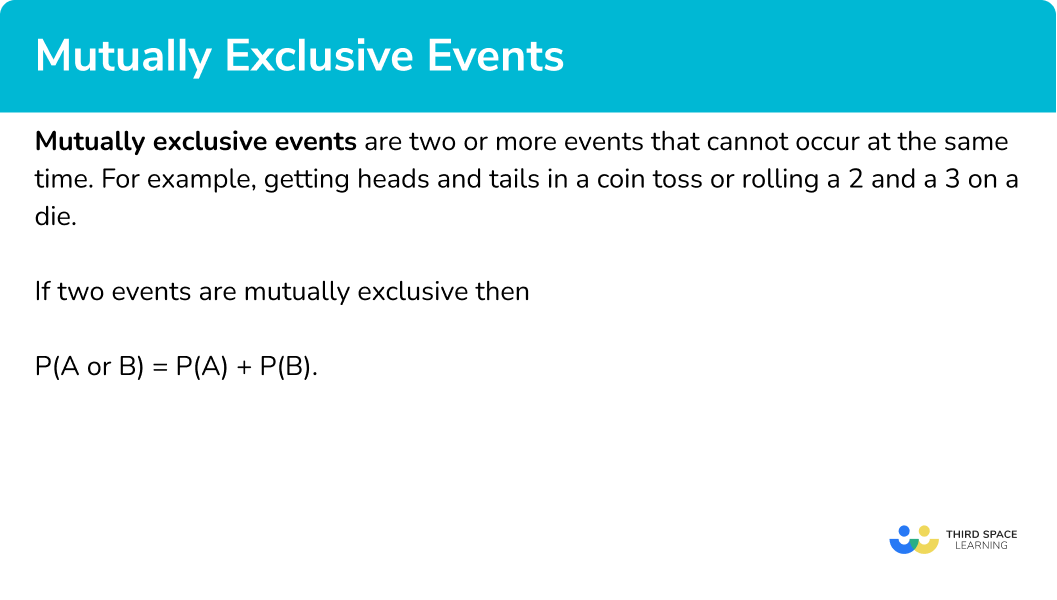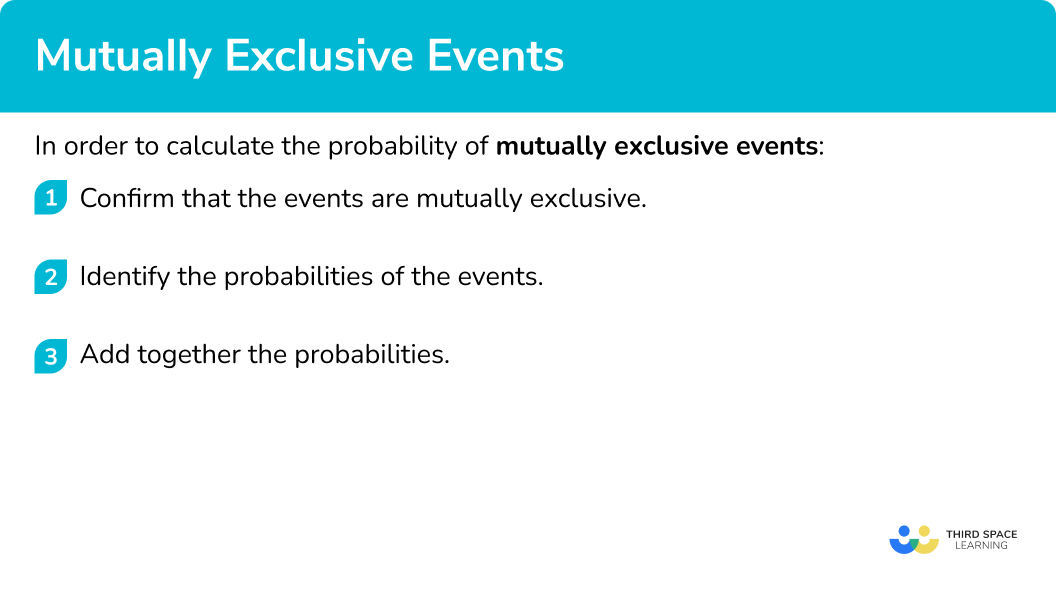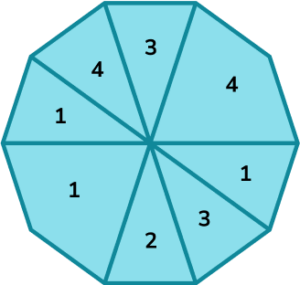# Mutually Exclusive Events

Here we will learn about mutually exclusive events, including what they are and how to find the probability of mutually exclusive events occurring.

There are also calculating probability worksheets based on Edexcel, AQA and OCR exam questions, along with further guidance on where to go next if you’re still stuck.

## What are mutually exclusive events?

Mutually exclusive events are two or more events that cannot occur at the same time. For example, getting heads and tails in a coin toss or rolling a 2 and a 3 on a die.

Mutually exclusive events are sometimes called disjoint events

If two events are mutually exclusive then

\bf{P(A} or \bf{B) = P(A) + P(B).}

This means that the probability of event A or event B occurring is equal to the probability of event A occurring, plus the probability of event B occurring.

We need to consider other probability rules within probability theory if two or more events are not mutually exclusive (can happen at the same time). Other types of events include,

• Independent events
• Dependent events
• Complementary events
• Exhaustive events

### What are mutually exclusive events?## How to calculate the probability of mutually exclusive events

In order to calculate the probability of mutually exclusive events:

1. Confirm that the events are mutually exclusive.
2. Identify the probabilities of the events.

### Explain how to calculate the probability of mutually exclusive events## Mutually exclusive events examples

### Example 1: exhaustive list of mutually exclusive events

A coin is flipped. Show that the probability of getting a heads or a tails is equal to 1.

1. Confirm that the events are mutually exclusive.

When flipping a coin, the two outcomes are heads or tails. These outcomes cannot occur at the same time and so the events are mutually exclusive.

2Identify the probabilities of the events.

The probability of a head is \cfrac{1}{2}.

The probability of a tail is \cfrac{1}{2}.

This is because the number of possible outcomes is 1, out of the total number of outcomes, 2 (heads or tails).

\cfrac{1}{2} + \cfrac{1}{2} = \cfrac{2}{2} = 1

The probability of getting a heads or a tails is 1.

Note: Such events are known as exhaustive as at least one of these events must occur when flipping a coin (heads or tails).

### Example 2: identifying mutually exclusive events

A fair six-sided die, numbered 1 to 6 is rolled. What is the probability of the die landing on a 3 or an even number?

As 3 is odd and the even numbers are 2 , 4 and 6 the events are mutually exclusive.

The probability of the die landing on 3 is \cfrac{1}{6} and the probability of it landing on an even number is \cfrac{3}{6} .

The probability of the die landing on a 3 or an even number is

\cfrac{1}{6}+\cfrac{3}{6}=\cfrac{4}{6}=\cfrac{2}{3}

### Example 3: probability of two mutually exclusive events

There are 3 red balls, 7 blue balls and 5 green balls in a bag. One ball is picked from the bag. Calculate the probability of a red or a blue ball being picked.

You cannot pick a red ball and blue ball at the same time therefore the events are mutually exclusive.

The probability of picking a red ball is \cfrac{3}{15}.

The probability of picking a blue ball is \cfrac{7}{15}.

\cfrac{3}{15} + \cfrac{7}{15} = \cfrac{10}{15} = \cfrac{2}{3}

The probability of picking a red ball or a blue ball is

\cfrac{10}{15} or \cfrac{2}{3}.

### Example 4: probability of three mutually exclusive events

The letters of the word M A T H E M A T I C S are written on identical blank cards. The cards are then shuffled an one is selected at random.

Calculated the probability of selecting the letter T or a vowel.

If you are selecting one card, you cannot pick a T and a vowel (A,E,I, O or U) at the same time so the events are mutually exclusive.

The probability of selecting a T is \cfrac{2}{11} .

The probability of selecting a vowel is \cfrac{4}{11} .

The probability of a T or a vowel being selected is

\cfrac{2}{11}+\cfrac{4}{11}=\cfrac{6}{11}.

### Example 5: calculating an individual probability

A biased die is numbered 1-6. On this die, the probability of rolling a 6 is \cfrac{1}{3} and the probability of rolling a 5 or a 6 is \cfrac{1}{2}. Work out the probability of rolling a 5.

You cannot roll a 5 and a 6 at the same time so the events are mutually exclusive.

The probability of rolling a 6 is \cfrac{1}{3}.

The probability of rolling a 5 or a 6 is \cfrac{1}{2}.

This time we know the probability of rolling a 6 and the probability of rolling a 5 or 6. If we write the probability of getting a 5 as P(5) then

\cfrac{1}{3} + P(5) = \cfrac{1}{2}.

Solving this by subtracting \cfrac{1}{3} from both sides, we have

P(5)=\cfrac{1}{2}-\cfrac{1}{3}=\cfrac{3}{6}-\cfrac{2}{6}=\cfrac{1}{6}.

The probability of rolling a 5 is \cfrac{1}{6}.

### Example 6: calculating an individual probability

There are black, white and blue socks only in a drawer. The probability of selecting a black sock from the drawer is 0.2 and the probability of selecting a white sock from the drawer is 0.45.

What is the probability of selecting a blue sock?

You cannot select a black, blue and white sock at the same time so the events are mutually exclusive.

The probability of selecting a black sock is 0.2.

The probability of selecting a white sock is 0.45.

Since picking a black, white or blue sock covers all possible outcomes, the events are exhaustive and therefore the total probability of selecting a black, a white or a blue sock is 1.

If we call the probability of selecting a blue sock P(B) then

0.2 + 0.45 + P(B) = 1.

Solving this, we have

\begin{aligned} 0.65+\text{P(B)}&=1\\\\ \text{P(B)}&=1-0.65\\\\ &=0.35 \end{aligned}

The probability of selecting a blue sock is 0.35.

### Common misconceptions

Fractions can only be added if they have a common denominator.

• Applying \bf{P(A} or \bf{B)=P(A)+P(B)} to non mutually exclusive events

For example, rolling a 3 and a prime number on a die. These events can happen at the same time since 3 is prime. Therefore they are not mutually exclusive and this formula does not work.

### Practice mutually exclusive events questions

1. Which of these events are not mutually exclusive?

Rolling a 3 and a 4 on a die.Rolling an even number and a 5 on a die.Rolling an even number and a prime number on a die.Rolling an even number and a 3 on a die.Mutually exclusive events cannot happen at the same time. 2 is an even number and a prime number so it is possible to roll an even number and a prime number at the same time, therefore the events are not mutually exclusive.

2. Which of these events are mutually exclusive?

Selecting a prime number and a factor of 12 from the numbers 1 to 12 .Selecting a consonant and a vowel from the alphabet.It raining and being sunny on a given day.Rolling a multiple of 2 and a multiple of  4 on a fair six-sided die.Mutually exclusive events cannot happen at the same time. Consonants and vowels cannot be selected at the same time.

3. In a bag there are 5 yellow balls, 6 blue balls, 7 red balls and 8 green balls. One ball is picked. What is the probability that a yellow or red ball is picked?

\cfrac{12}{26}\cfrac{5}{7}\cfrac{14}{26}\cfrac{13}{26}There are 26 balls altogether.

The probability of picking a yellow ball is \cfrac{5}{26}.

The probability of picking a red ball is \cfrac{7}{26}.

The probability of picking a yellow or red ball is \cfrac{5}{26}+\cfrac{7}{26}=\cfrac{12}{26}.

4. What is the probability of the following spinner landing on a 1, 2 or 3?\cfrac{6}{8}\cfrac{3}{4}\cfrac{7}{10}\cfrac{3}{10}The spinner has 10 sides.

The probability of landing on a 1 is \cfrac{4}{10}.

The probability of landing on a 2 is \cfrac{1}{10}.

The probability of landing on a 3 is \cfrac{2}{10}.

The probability of landing on a 1, 2 or 3 is \cfrac{4}{10}+\cfrac{1}{10}+\cfrac{2}{10}=\cfrac{7}{10}.

5. Lucy has a box of chocolates containing milk, white and dark chocolates. The probability of picking a milk chocolate from the box is \cfrac{1}{2} and the probability of picking a milk chocolate or a white chocolate is \cfrac{4}{5}.

What is the probability of picking a white chocolate from the box?

\cfrac{3}{10}\cfrac{13}{10}\cfrac{3}{3}\cfrac{7}{10}The events are mutually exclusive therefore

P(A or B)=P(A)+P(B).

\begin{aligned} \cfrac{4}{5}&=\cfrac{1}{2}+P(W)\\\\ \cfrac{4}{5}-\cfrac{1}{2}&=P(W)\\\\ P(W)&=\cfrac{8}{10}-\cfrac{5}{10}\\\\ &=\cfrac{3}{10} \end{aligned}

6. The probability that Hannah will be early to school is 0.3. The probability that Hannah will be on time to school is 0.5. What is the probability Hannah will be late to school?

0.80.70.20.1The events are mutually exclusive and the only possibilities are early, on time and late which means the sum of the probabilities must be 1.

\begin{aligned} 0.3+0.5+P(L)&=1\\\\ P(L)&=0.2 \end{aligned}

### Mutually exclusive events GCSE questions

1. Barry rolls a fair 6 sided die. What is the probability Barry rolls an odd number or a 6?

(3 marks)

P(\text{Odd})=\cfrac{3}{6}

(1)

P(6)=\cfrac{1}{6}

(1)

P(Odd \ or \ 6)= \cfrac{3}{6}+\cfrac{1}{6}=\cfrac{4}{6}= \cfrac{2}{3}

(1)

2. (a) Three coins are tossed. By listing all of the possible outcomes, identify the total number of possible outcomes.

(b) Use your list of outcomes to calculate the probability of getting a head on the first coin followed by either two heads or two tails.

(5 marks)

(a)

Six of the outcomes listed.

(1)

All 8 outcomes listed – HHH, HTH, HHT, HTT, TTT, THT, TTH, THH.

(1)

8 outcomes stated.

(1)

(b)

P(HHH)=\cfrac{1}{8} and P(HTT)=\cfrac{1}{8}

(1)

\cfrac{1}{8} + \cfrac{1}{8} = \cfrac{2}{8} or \cfrac{1}{4}

(1)

3. (a) A lucky dip contains 4 types of presents. The probability of choosing each type of present is shown below.What is the probability of choosing a pencil or a yoyo?

(b) What is the probability of choosing a notepad?

(3 marks)

(a)

0.4 + 0.3 = 0.7

(1)

(b)

0.4 + 0.15 + 0.3 = 0.85

(1)

1 – 0.85 = 0.15

(1)

## Learning checklist

You have now learned how to:

• Identify mutually exclusive events
• Calculate the probability of mutually exclusive events occuring

## Still stuck?

Prepare your KS4 students for maths GCSEs success with Third Space Learning. Weekly online one to one GCSE maths revision lessons delivered by expert maths tutors.

Find out more about our GCSE maths tuition programme.